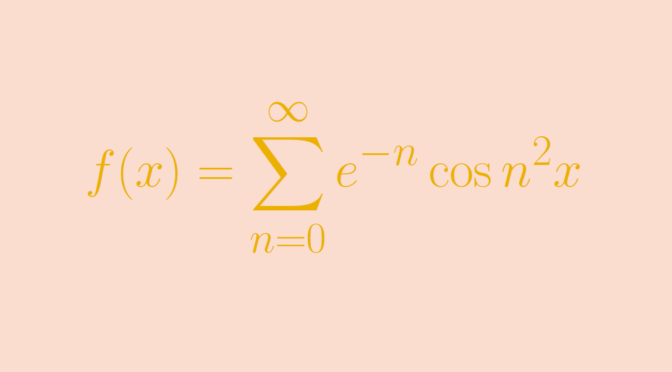# A function whose Maclaurin series converges only at zero

Let’s describe a real function $$f$$ whose Maclaurin series converges only at zero. For $$n \ge 0$$ we denote $$f_n(x)= e^{-n} \cos n^2x$$ and $f(x) = \sum_{n=0}^\infty f_n(x)=\sum_{n=0}^\infty e^{-n} \cos n^2 x.$ For $$k \ge 0$$, the $$k$$th-derivative of $$f_n$$ is $f_n^{(k)}(x) = e^{-n} n^{2k} \cos \left(n^2 x + \frac{k \pi}{2}\right)$ and $\left\vert f_n^{(k)}(x) \right\vert \le e^{-n} n^{2k}$ for all $$x \in \mathbb R$$. Therefore $$\displaystyle \sum_{n=0}^\infty f_n^{(k)}(x)$$ is normally convergent and $$f$$ is an indefinitely differentiable function with $f^{(k)}(x) = \sum_{n=0}^\infty e^{-n} n^{2k} \cos \left(n^2 x + \frac{k \pi}{2}\right).$ Its Maclaurin series has only terms of even degree and the absolute value of the term of degree $$2k$$ is $\left(\sum_{n=0}^\infty e^{-n} n^{4k}\right)\frac{x^{2k}}{(2k)!} > e^{-2k} (2k)^{4k}\frac{x^{2k}}{(2k)!} > \left(\frac{2kx}{e}\right)^{2k}.$ The right hand side of this inequality is greater than $$1$$ for $$k \ge \frac{e}{2x}$$. This means that for any nonzero $$x$$ the Maclaurin series for $$f$$ diverges.